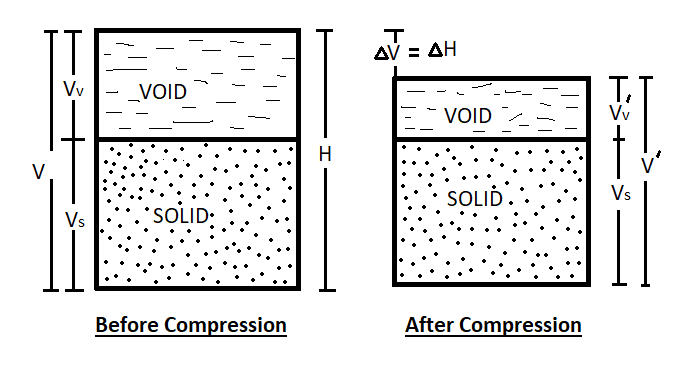# Consolidation Settlement Analysis – Soil

5th May 2019

## Consolidation Settlement Analysis

In one dimensional consolidation, the change in the height(ΔH) (i.e settlement) per unit of original height(H) equals to the change in volume(ΔV) per unit of the original volume(V).
ΔH/H = ΔV/V ………….(1).

Now, at an initial condition, void ratio(e0) = Vv/Vs Or Vv= e0.Vs

Where Vv and Vs are the volume of voids and volume of soil solids respectively.
But, V = Vv + Vs
Or, V = e0.Vs+ Vs
Or, V = Vs(1+e0)
At compressed condition, void ratio(e) = v/Vs
Where V՛v is the volume of voids at compressed condition.
Now, V՛ = Vs(1+e)
Where V՛ and e are the volume of soil sample and void ratio respectively at the compressed condition.

Therefore, change in volume(ΔV) = V – V՛
Or, ΔV = Vs(1+e0) – Vs(1+e)
Or, ΔV = Vs(e0-e)
Or, ΔV = Vs.Δe Where [Δe (e0-e)]
Now, ΔV/V = Vs.Δe/V
Or, ΔV/V = Vs.Δe/Vs(1+e0)
Or, ΔV/V = Δe/(1+e0) ……………(2).

From compression curve of e versus Log p we get
Compression Index(Cc) = Δe/ Log10 (p/p0)
Or, Δe = CLog10 (p/p0) ………………(3).
Where p0 is the initial pressure and p is the pressure at the compressed condition.Now, from equation (1), (2), and (3) we get
ΔH/H = ΔV/V
Or, ΔH = (ΔV/V).H
Or, ΔH = {Δe/(1+e0)}.H
Or, ΔH = {CLog10 (p/p0)/(1+e0)}.H

### The Equation of The Consolidation Settlement

• ΔH = {CLog10 (p/p0)/(1+e0)}.H